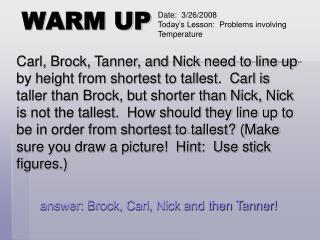# WARM UP - PowerPoint PPT PresentationDownload PresentationWARM UP

WARM UP
Download Presentation## WARM UP

- - - - - - - - - - - - - - - - - - - - - - - - - - - E N D - - - - - - - - - - - - - - - - - - - - - - - - - - -
##### Presentation Transcript

1. Date: 3/26/2008 Today’s Lesson: Problems involving Temperature WARM UP Carl, Brock, Tanner, and Nick need to line up by height from shortest to tallest. Carl is taller than Brock, but shorter than Nick, Nick is not the tallest. How should they line up to be in order from shortest to tallest? (Make sure you draw a picture! Hint: Use stick figures.) answer: Brock, Carl, Nick and then Tanner!

2. TAKS WARM UP

3. TODAY’S OBJECTIVE • Today you will learn how to solve problems involving temperature. Why is it so cold in here?

4. LAUNCH Please take down the following information for your notes. • You can use customary or metric units to measure temperature. • Fahrenheit (°F) is the customary unit for measuring temperature. • Celsius (°C) is the metric unit for measuring temperature.

5. PROBLEM A thermometer shows the temperature at 6:00 a.m. to be [39°F and 4°C]. The temperature rises 9 degrees by 8 a.m. What is the temperature in degrees Celsius at 8 a.m.?

6. STEP 1 PROBLEM A thermometer shows the temperature at 6:00 a.m. to be [39°F and 4°C]. The temperature rises 9 degrees by 8 a.m. What is the temperature in degrees Celsius at 8 a.m.? On the Celsius scale, each tick mark stands for 1 degree. How many tick marks above 0°C is the temperature?______ What is the temperature at 6:00 a.m.? _______°C 4 4

7. STEP 2 PROBLEM A thermometer shows the temperature at 6:00 a.m. to be [39°F and 4°C]. The temperature rises 9 degrees by 8 a.m. What is the temperature in degrees Celsius at 8 a.m.? To find the temperature at 8 a.m., add the amount of increase, 9 degrees, to the temperature, at 6:00 a.m. 4 + 9 = ________ Write the temperature using the correct unit of measure. The temperature is ______. 13 13°

8. SOLUTION PROBLEM A thermometer shows the temperature at 6:00 a.m. to be [39°F and 4°C]. The temperature rises 9 degrees by 8 a.m. What is the temperature in degrees Celsius at 8 a.m.? What was the temperature at 8 a.m.? _______________________________________ 13°C SOLUTION

9. EXPLORE • Complete Lesson 40, Solve Problems Involving Temperature #s 1-5 with a partner (Worksheet). • Be prepared to read and discuss your answers with the class

10. SUMMARIZE • What have you learned today about solving problems involving temperature? • What helpful strategies did you use to solve problems 1-5?

11. SUMMARIZE cont. A thermometer shows the temperature at 6:00 a.m. to be [39°F and 4°C]. The temperature rises 9 degrees by 8 a.m. What is the temperature in degrees Celsius at 8 a.m.? • If we looked back at the previous problem, what would be the temperature at 8:00 a.m. in degrees Fahrenheit? 48°F

12. PRACTICE • MEASURING UP – LEVEL F • LESSON 40 • PAGE 170 • SOLVE PROBLEMS INVOLVING TEMPERATURE • REMAINING QUESTIONS 6-8